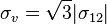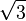# Single shear element in stress tensor: Finding Von Mises

jasc15
When finding the Von Mises of given a stress tensor who's only element is a single shear component (τ):

\begin{bmatrix}
0 & τ & 0\\
τ & 0 & 0\\
0 & 0 & 0
\end{bmatrix}
the result is simply √3×τ. Is the Von Mises criterion not valid when considering a single component as in this example? I can't seem to reconcile that a calculated shear stress (say by a simple shaft twisting where τ=Tc/J) should be multiplied by √3. I understand that when using the Von Mises yield criterion, it is to be compared to the uniaxial yield allowable, not the shear allowable. However, the yield allowable is not √3 greater than the shear allowable.

In the example I am actually considering there is also normal component, but I want to see the effect of the two components in isolation as well as combined

Gold Member
I think this is consistent with the calculations of the Von Mises criterion. In the case of a pure shear stress,according to the load scenario table in the page below.

See below information from Wikipedia:
https://en.wikipedia.org/wiki/Von_Mises_yield_criterion

Wikipedia.org said:
In the case of pure shear stress, [PLAIN]https://upload.wikimedia.org/math/4/4/d/44dcfff99c0c0c4fc2ab5177bb18be1f.png, [Broken] while all other [PLAIN]https://upload.wikimedia.org/math/2/5/d/25d584aefa115a4f46e0d761fef717d8.png, [Broken] von Mises criterion becomes:

This means that, at the onset of yielding, the magnitude of the shear stress in pure shear istimes lower than the tensile stress in the case of simple tension.

Last edited by a moderator:
jasc15
Thanks for the reply. How am I to understand this from the point of view of a simple strength of materials problem, where I am asked to find the shear stress in a shaft with an applied torque, and to compare it to the shear allowable for that material.

For example, a torque of 150 in*lb and a shaft radius of 0.15 in results in an applied shear stress of

τ = T*c/J = 150*0.15/(Π*.152) = 28,294 psi.

The shear allowable of the material I am working with is 31000 psi, and the uniaxial yield allowable is 36000 psi.

Using the simple shear stress calculated, I get a margin of safety of 31,000/28,294 -1 = 0.10

Using Von Mises, I get √3*28,294 psi = 49,007 psi, with a resulting margin of 36,000/49,007 -1 = -0.27

Is this simply a matter of choosing how conservative you want to be in design? I wouldn't be comfortable using only the first method knowing the second method results in negative margin.